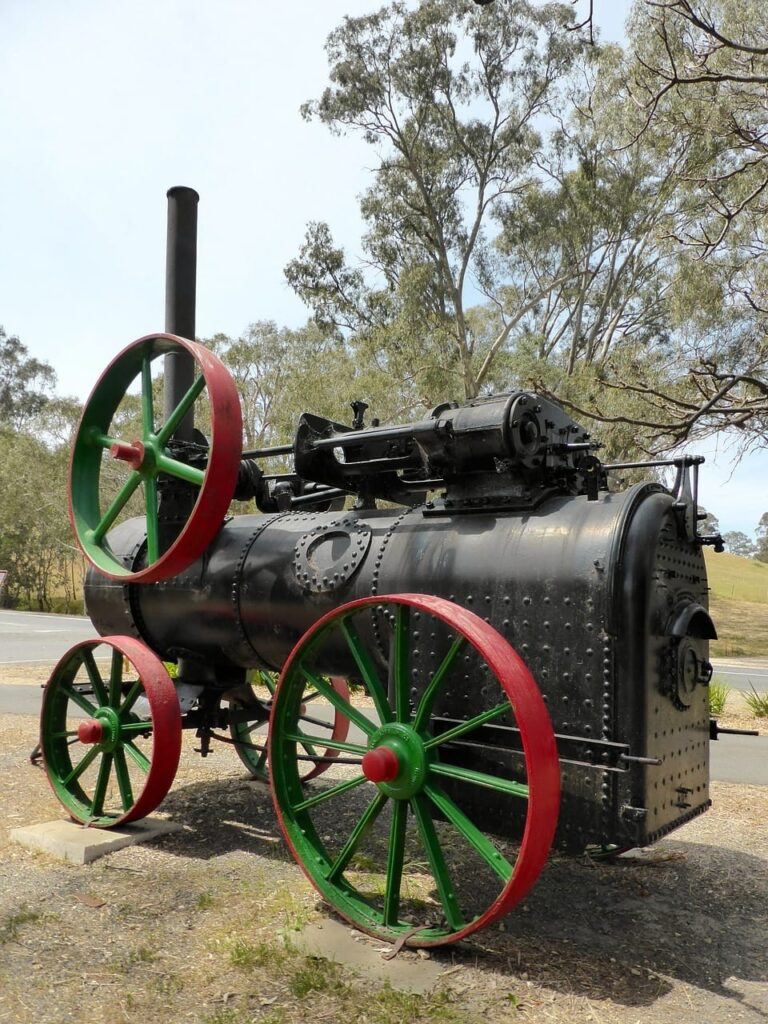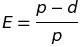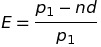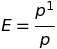# How To: Successfully Determine Ligament Calculations

How To: Successfully Determine Ligament Calculations was a topic I initially found very difficult while studying for 2A1.

While helping other Power Engineers study for their exam, I’ve found out many of them struggle with this topic as well.

If your struggling with ligament calculations, I’m sure this post and the seven practice questions below, will improve your understanding.

I recommend the following before continuing further or completing the practice questions below

1. Review the following practice questions for PG-27.2.2 page 10:

How To: Determine The Minimum Required Thickness Of A Header

How To: Determine The Maximum Allowable Working Pressure Of A Header

2. The examples provided in the 2007 ASME Boiler & Pressure Vessel code on page 28 from PG-52.1 to PG-52.5. They will definitely improve your understanding of how to complete ligament calculations and the seven practice questions below, much easier to complete.

All referenced page numbers are from the 2007 ASME Boiler & Pressure Vessel Code

## Formulas

PG-52.2.1 page 28 When the pitch of the tubes on every tube row is equal (as in Fig. PG-52.2), the equation is:PG-52.2.2 page 28 When the pitch of the tube holes on any one row is unequal (as in Fig. PG-52.3 or Fig. PG-52.4), the equation is:PG-52.4 Holes Along a Diagonal. page 28 When a shell or drum is drilled for tube holes as shown in Fig. PG-52.5 the efficiency of these ligaments shall be that given by the diagram in Fig. Pg-52.1. The abscissa and the ratio shall be computed.

Abscissa Equation:Ratio Equation:This paragraph is referencing Fig. PG-52.1 page 29 With these values the efficiency may be read off the ordinate. Should the point fall above the curve of equal efficiency “Point B” for the diagonal and longitudinal ligaments, the longitudinal ligaments will be the weaker, in which case the efficiency is computed from the following equation:## Formula Variables

The variables used in the formulas provided above to are defined in PG-52.6 page 30 as follows:

d = diameter of openings, in. (mm)

E = efficiency of ligament

p = longitudinal pitch of adjacent openings, in. (mm)

p’ = diagonal pitch of adjacent openings, in. (mm)

p‚ = pitch between corresponding openings in a series of symmetrical groups of openings, in. (mm)

n = number of openings in length p‚

The pitch shall be measured either on the flat plate before rolling or on the median surface after rolling.

[table id=1 /]

## How To: Successfully Determine Ligament Calculations Questions

Note: All code questions are to be calculated in (mm) and (MPa) unless otherwise stated. Convert accordingly and properly before the calculation.

## Summary

One paragraph I have found particularly important to be aware of when learning how to: successfully determine ligament calculations is:

PG-52.3 Openings Transverse to Vessel Axis. The strength of those ligaments between the tube holes that are subjected to a longitudinal stress shall be at least one-half the required strength of those ligaments that come between the tube holes that are subjected to a circumferential stress.

The requirements of PG-52.3 show up in question #51.

Good luck!

Robbie

Power Engineering 101

### 6 thoughts on “How To: Successfully Determine Ligament Calculations”

1.I had a question similar to 2A1 Question 50, but the final part asked if the longitudinal and circumferential ligaments met the requirement of PG-52.3. I am unsure how to respond to this question. All examples of ‘strength’ that I have seen involved diagonal numbers which the question did not provide.

2.I recently had the following question come up in my studying.

” A power boiler drum has tubes attached to it in rows with longitudinal separation of 550mm, and circumferentially separated by 12 degrees. The tube hole diameter is 78mm, and the inside radius of the vessel is 660mm with a shell thickness of 18mm. Max stress is 112 MPA. Find the design pressure of the vessel. ”

Now my problem with this question is: which efficiency to use in the final design pressure calculation?

The calculated Efficiency for longitudinal is 0.858, while for circumferential is 0.451.

I am struggling to find an answer in the code book with regards to this sort of situation. My instinct is to use the lower efficiency in order to lower the final MAWP, but the answer key uses the longitudinal efficiency of 0.858, while quoting PG-52.3. Their interpretation of PG 52.3 was that the longitudinal efficiency is used in the equation if the circumferential efficiency is less than half of longitudinal.

I am struggling to find a reference for which Efficiency to use in this sort of question.

1.I believe they use the longitudinal stress because circumferential stress is 2x the longitudinal stress. meaning .451×2=.902, giving the longitudinal stress the lesser of the two.

1.If the Circ efficiency (doubled) is still the lowest efficiency. Do you use the actual stated efficiency or the 2X version?? this the only part I don’t get (Ignoring the latest ASME Sect I pg 52.3 which is a whole new ball game)

1.Here’s what I understand: the circumferential efficiency equivalent to longitudinal efficiency = longitudinal efficiency divided by 2

E long. = E circ. x 2

3.How to determine ligament efficiency in a distribution header where nozzles of unequal diameter and unequal pitch?

## Enter Your Email To Get Access To The Free Training!error: Alert: Content is protected !!